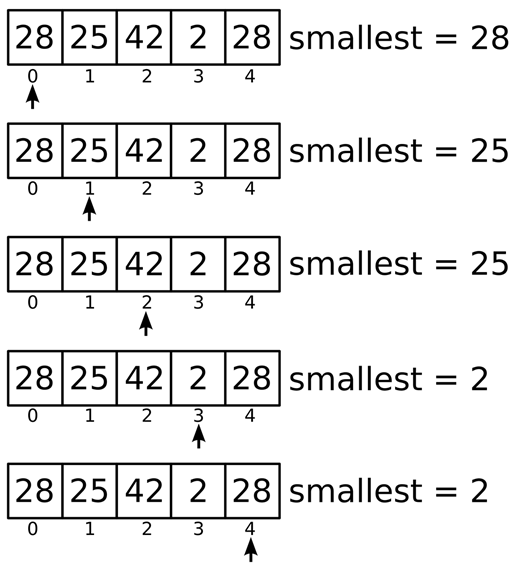# Exercise #12: Smallest & Biggest

getSmallest([28, 25, 42, 2, 28])   2Python’s built-in `min()` and max() functions return the smallest and biggest numbers in a list of numbers passed, respectively. In this exercise, you’ll reimplement the behavior of these functions.

This is the sort of problem that is trivial for a human to solve by hand if the list of numbers is short. However, if the list contains thousands, millions, or billions of numbers, you’ll need to program a computer to perform the calculation.

Exercise Description

Write a `getSmallest()` function that has a `numbers` parameter. The `numbers` parameter will be a list of integer and floating-point number values. The function returns the smallest value in the list. If the list is empty, the function should return `None`. Since this function replicates Python’s `min()` function, your solution shouldn’t use it.

These Python `assert` statements stop the program if their condition is `False`. Copy them to the bottom of your solution program. Your solution is correct if the following `assert` statements’ conditions are all True:

assert getSmallest([1, 2, 3]) == 1

assert getSmallest([3, 2, 1]) == 1

assert getSmallest([28, 25, 42, 2, 28]) == 2

assert getSmallest() == 1

assert getSmallest([]) == None

Try to write a solution based on the information in this description. If you still have trouble solving this exercise, read the Solution Design and Special Cases and Gotchas sections for additional hints.

When you are done with this exercise, write a getBiggest() function which returns the biggest number instead of the smallest number.

Prerequisite concepts: `len()`, for loops, lists, `None` value

Solution Design

Think about how you would solve this problem without a computer, given a list of numbers written on paper. You would use the first number as the smallest number, and then read every number after it. If the next number is smallest than the smallest number you’ve seen so far, it becomes the new smallest number. Let’s look at a small example. Figure 12-1 shows how looping over the list `[28, 25, 42, 2, 28]` would affect the contents of a variable named `smallest` that tracks the smallest number seen so far.Figure 12-1: The value in smallest contains the smallest integer found so far as the `for` loop iterates over the list `[28, 25, 42, 2, 28]`.

Creates a variable named `smallest` to track the smallest value found so far and set it to the first value in the list to start. Then have a `for` loop that loops over every number in the list from left to right, and if the number is less than the current value in `smallest`, it becomes the new value in `smallest`. After the loop finishes, the function returns the smallest value.

Special Cases and Gotchas

The function should first check if the list is empty. In that case, return `None`. And by starting the smallest variable to the first number in a non-empty numbers list, you can guarantee that `smallest` is always initialized to a value from the list.

Now try to write a solution based on the information in the previous sections. If you still have trouble solving this exercise, read the Solution Template section for additional hints.

Solution Template

Try to first write a solution from scratch. But if you have difficulty, you can use the following partial program as a starting place. Copy the following code from https://invpy.com/smallest-template.py and paste it into your code editor. Replace the underscores with code to make a working program:

def getSmallest(numbers):

# If the numbers list is empty, return None:

if len(____) == ____:

return None

# Create a variable that tracks the smallest value so far, and start

# it off a the first value in the list:

smallest = numbers[____]

# Loop over each number in the numbers list:

for number in ____:

# If the number is smaller than the current smallest value, make

# it the new smallest value:

if ____ < smallest:

____ = number

# Return the smallest value found:

____ smallest

The complete solution for this exercise is given in Appendix A and https://invpy.com/smallest.py. You can view each step of this program as it runs under a debugger at https://invpy.com/smallest-debug/.

The benefit of writing a computer program to do a simple task like finding the smallest number in a list is that a computer can process a list of millions of numbers in seconds. We can simulate this by having the computer generate one million random numbers in a list, and then pass that list to our `getSmallest()` function. On my computer, this program takes a few seconds, and most of that time is spent displaying the million numbers on the screen.

Write the following program and save it in a file named testsmallest.py. Run it from the same folder as your smallest.py file so that it can import it as a module:

import random, smallest

numbers = []

for i in range(1000000):

numbers.append(random.randint(1, 1000000000))

print('Numbers:', numbers)

print('Smallest number is', smallest.getSmallest(numbers))

When run, this program displays the million numbers between 1 and 1,000,000,000 it generated, along with the smallest number in that list.